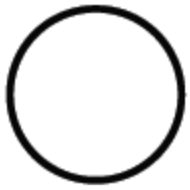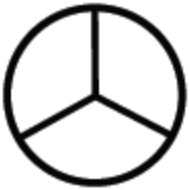### Home > CC2MN > Chapter 1 > Lesson 1.1.5 > Problem1-47

1-47.

Represent each of the fractions below both with a diagram and with words.

1. $\frac{2}{3}$

The denominator (below the fraction bar) shows how many sections there are in the diagram. The numerator (above the fraction bar) shows how many of those sections are filled in.

Draw a shape. An unshaded circle

Divide the shape into equal sections. Since the denominator is $3$, divide the shape into $3$ sections of equal sizes. Circle is divided into 3 pie shaped pieces.

Since the numerator is $2$, fill in $2$ of those $3$ sections you created. 2 of the 3 pie shaped pieces are shaded.2. $1\frac{1}{8}$

Read the number aloud. One AND one eighth means that you have one whole diagram and one eighth of another. Now try drawing your own diagram.

3. $\frac{6}{9}$

Refer to part (a) for help.